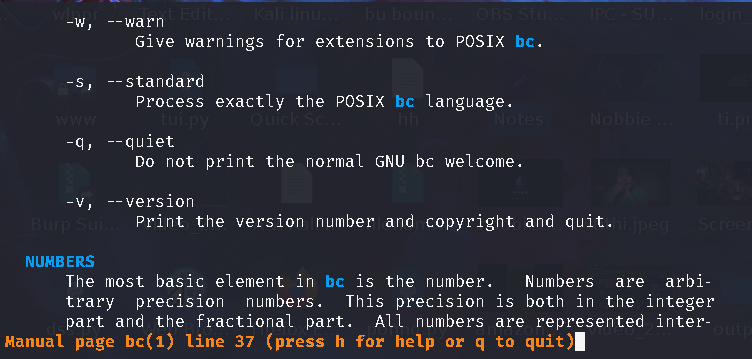# How to Use GNU bc (Basic Calculator) in Linux

• Last Updated : 30 Jun, 2021

The Basic calculator (bc) is an arbitrary-precision calculator that you can use as a simple scientific or financial calculator on the command-line interface of your Linux system. The syntax is similar to the C programming language. You just have to type the following command to see whether bc is already present in your system or not.

`bc `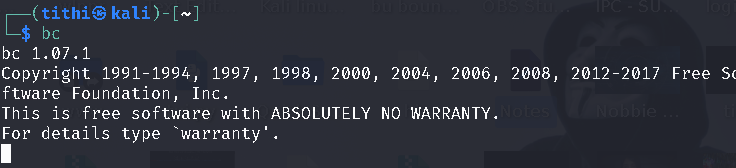If you are a Debian/Ubuntu-based Linux user and didn’t find bc in your system, then you can use the command below to install bc:

`\$ sudo apt install bc    `

### How to use?

If you are already done with the installation or you already have it on your system then just type bc in your terminal and start calculating whatever you want to

`\$ bc  `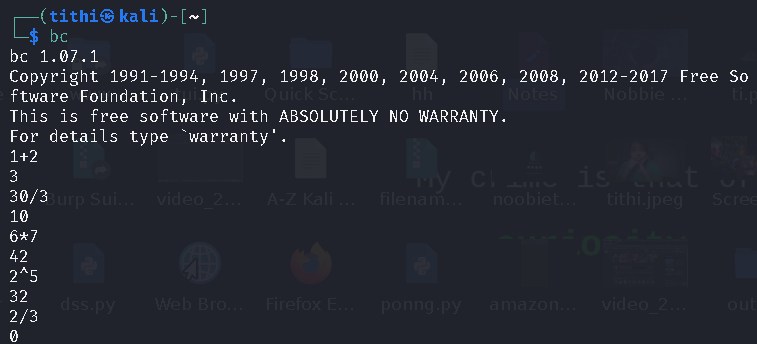Here we can see that bc is working with arbitrary precision and it is returning 0 when a decimal is coming while dividing 2/3.

#### -l option:

The -l option after bc will set the  default scale  i.e digits after the decimal point to 20 and adds several additional mathematical functions to the language.

`\$ bc  -l`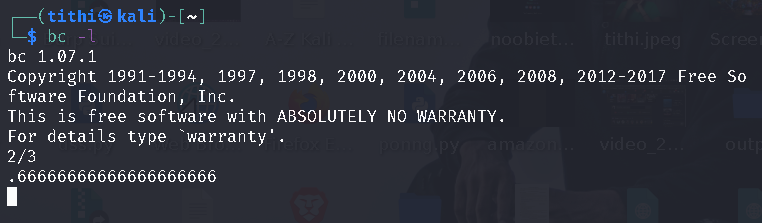Alternatively, you can also specify the scale value, by which you can set the limit of the digits after the decimal point according to your wish which is not necessarily be 20 like the previous one.

`scale=3; 2/3`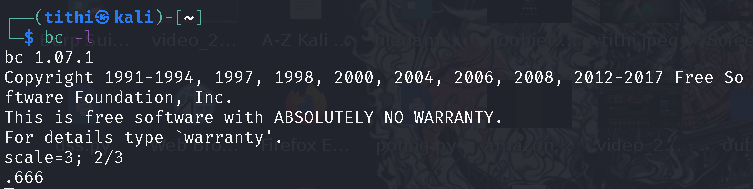You can also use bc directly in the command line shells like zsh and bash by passing the arguments to bc like this:

```\$  bc -l <<< "scale=3 ; 2/3"
\$  echo 'scale=3;2/3' | bc ```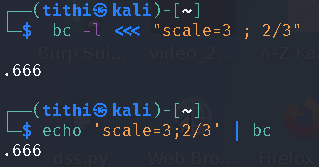### Bc Functions Using mathlib:

Just like the scale function, you can perform other advanced functions of maths too, such as sin, cos, tan, log functions by using the standard math library provided by bc, which can be used with the help of the command-line option –mathlib or -l with bc.

```Syntax

s (x) for sine
c (x) for cosine
a (x) for arctangent
l (x) for logarithm
e (x) for exponential function
sqrt(x) for square root```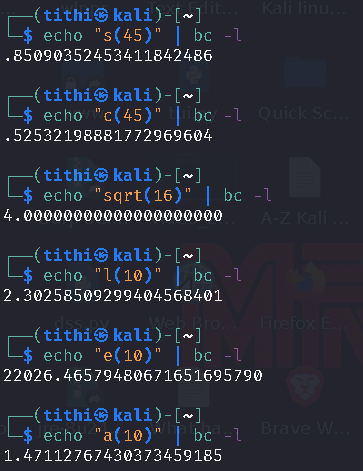### Make custom Bc Functions:

Till now, we have discussed the built-in functions of the basic calculator, but you can also create your own functions according to your requirements. To make your own custom bc function, you have to start with define keywords. The syntax is as follows:-

```define function_name ( parameters ) {
statement...
return statement;
}```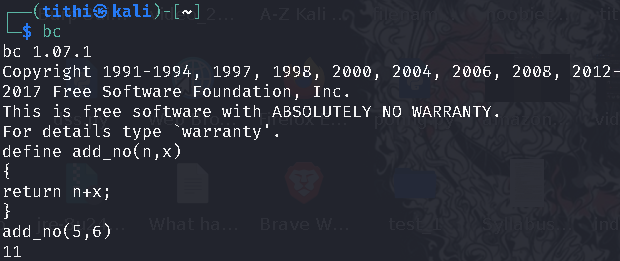### Convert Binary To Decimal And Decimal To Binary Using BC:

The variables ibase and obase you can convert binary to decimal and vice-versa.

Convert binary to decimal:

`\$ echo 'ibase=2;obase=A;11' | bc -l`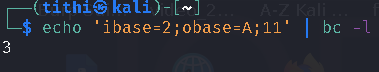Convert decimal to binary:

`\$ echo 'ibase=10;obase=2;3' | bc`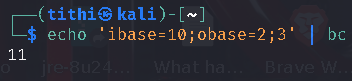### Take Input From A File:

Typing expression each time could be hectic, so you can put all of your maths expressions that you want to execute in a  single file and execute them using the bc command.

`bc -q filename`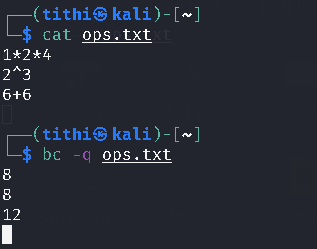You can take an idea from everything explained here and use it to create several shell scripts to automate your work.

`\$ man bc`